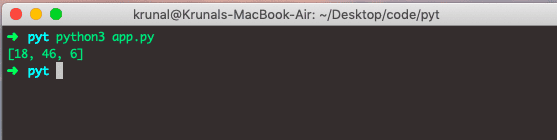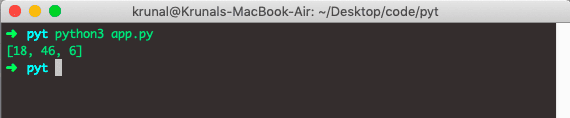# Python filter: The Complete Guide

The filter() method filters the given iterable with the help of a function that tests each item in the iterable to be true or not. Python filter() method constructs the iterator from items of an iterable for which a function returns True.

## Python filter

Python filter() is a built-in function that returns an iterator where the elements are filtered through a function to test whether an element is accepted.

### Syntax

`filter(function, iterable)`

### Parameter

The function parameter is to be run for each item in the iterable. The function that tests if elements of the iterable return True or False. If None, the function defaults to the Identity function, which returns False.

An iterable is the parameter to be filtered. An iterable which is to be filtered could be a Python Data Structure like setlisttuple, or container of any iterators.

See the following example of the Python Filter method.

```# app.py

nums = [21, 19, 18, 46, 6, 29]
def checkEven(x):
if x % 2 == 0:
return True
else:
return False

data = filter(checkEven, nums)
print(list(data))
```

In the above code, we have defined the list and function, which takes one parameter and check that item if it is even. If it is True, it will return true, and we will get that item in the data object, and then we can convert it to the list.

The filter() method then passes each integer to the checkEven() method to check if it returns True or False. Ultimately, it creates the iterator of the ones that return true.

See the output below.## Lambda and filter() function in Python

We can use the built-in lambda function inside the Python filter() to find all the numbers divisible by 2 on the list. In Python, an anonymous function means that a function is without a name. See the below example of a filter method with an anonymous function.

If we use the Lambda function, then the size of the code compared to the above code is decreased to 3 lines. See the below code.

```# app.py

integers = [21, 19, 18, 46, 6, 29]
even = list(filter(lambda x: x % 2 ==  0, integers))
print(even)```

Now, run the file and see the output.The filter() method returns the iterator that passed the function check for each element in the iterable. The returned elements are those who passed the test.

That’s it for this tutorial.

This site uses Akismet to reduce spam. Learn how your comment data is processed.